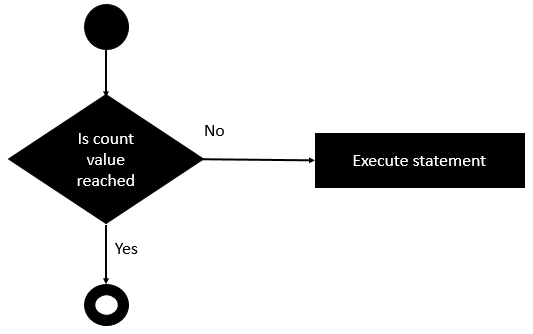# Rexx - do Loop

The do loop is used to execute a number of statements for a certain number of times. The number of times that the statement needs to be executed is determined by the value passed to the do loop.

## Syntax

The syntax of the do loop statement is as follows −

```do count
statement #1
statement #2
...
End
```

Statement#1 and Statement#2 are a series of a block of statements that get executed in the do loop. The count variable symbolizes the number of times the do loop needs to be executed.

## Flow Diagram

The following diagram shows the diagrammatic explanation of this loop.The key point to note about this loop is that the do loop will execute based on the value of the count variable.

The following program is an example of a do loop statement.

## Example

```/* Main program */
do 5
say "hello"
end
```

The following key points need to be noted about the above program −

• The count in the above case is 5. So the do loop will be run for 5 times.

• In the do loop, the phrase hello is displayed to the console.

The output of the above program will be −

```hello
hello
hello
hello
hello
```
rexx_loops.htm